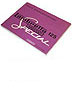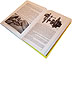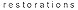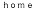### Vespa Serial Number Locations

Vespa Large Frame - 70's onward (& 60s bikes with removable left hand side cowls)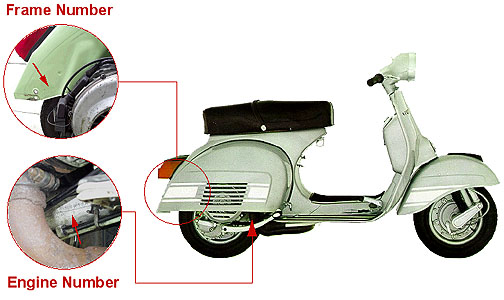Frame: The engine side cowl must be removed to see the frame serial number. It is stamped to the right of the hole for the cowl pin. The number will start with a prefix (ex. VSX1T) which denotes the model, then a star and a 4 to 6 digit number with a star at the end which denotes the year. A typical frame number may look like this: VSX1T*123456*

Engine: Look on the engine swingarm to see the engine serial number. It is stamped on the cast aluminum swingarm right behind the exhaust stub on the barrel. The number will start with a prefix (ex. VSE1M) which denotes the model, then a star and a 4 to 6 digit number with a star at the end which denotes the year. A typical engine number may look like this: VSE1M*123456*

Vespa Small Frame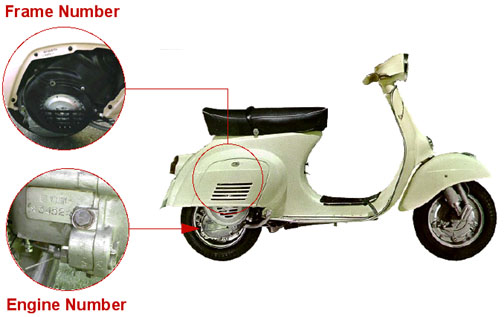Frame: The engine side door must be removed to see the frame serial number. It is stamped on the upper lip where the catch engages. The number will start with a prefix (ex. V9A1T) which denotes the model, then a star and a 4 to 6 digit number with a star at the end which denotes the year. A typical frame number may look like this: V9A1T*123456*

Engine: Looking at the rear of the bike, look on the rear face of the engine casing to see the engine serial number just to the left of the transmission oil fill hole. The number will start with a prefix (ex. V9A1M) which denotes the model, then a star and a 4 to 6 digit number with a star at the end which denotes the year. A typical engine number may look like this: V9A1M*123456*

Vespa Large Frame - 60's - 70's (with fixed left hand panel glovebox)Frame: The left hand side cowl is attached on these models and the frame serial number is stamped on the frame at the underside of the side cowl where it hits the body, above the rear wheel. The number will start with a prefix (ex. VBA1T) which denotes the model, then a star and a 4 to 6 digit number with a star at the end which denotes the year. A typical frame number may look like this: VBA1T*123456*

Engine: Look on the engine swingarm to see the engine serial number. It is stamped on the cast aluminum swingarm right behind the exhaust stub on the barrel. The number will start with a prefix (ex. VSE1M) which denotes the model, then a star and a 4 to 6 digit number with a star at the end which denotes the year. A typical engine number may look like this: VBA1M*123456*

Vespa "Wide Mount" Frame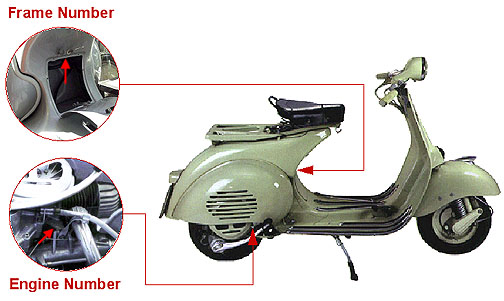Frame: The small door in the frame below the seat must be opened to see the frame serial number. It is stamped to the upper lip of the opening just below the choke and fuel levers. The number will start with a prefix (ex. VS51T) which denotes the model, then a star and a 4 to 6 digit number with a star at the end which denotes the year. A typical frame number may look like this: VS51T*123456*

Engine: Look on the engine swingarm to see the engine serial number. It is stamped on the cast aluminum swingarm right behind the exhaust stub on the barrel. The number will start with a prefix (ex. VS51M) which denotes the model, then a star and a 4 to 6 digit number with a star at the end which denotes the year. A typical engine number may look like this: VS51M*123456*